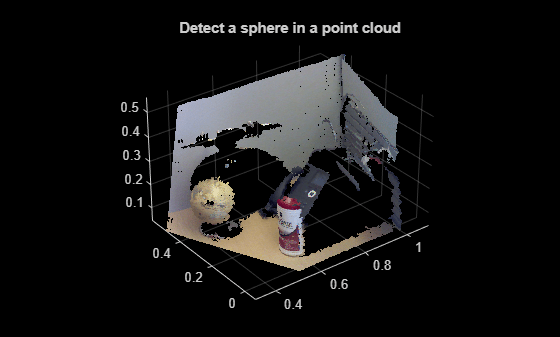# pcfitsphere

Fit sphere to 3-D point cloud

## Syntax

``model = pcfitsphere(ptCloudIn,maxDistance)``
``````[___,inlierIndices,outlierIndices] = pcfitsphere(___)``````
``````[___,meanError] = pcfitsphere(___)``````
``[___] = pcfitsphere(___,Name=Value)``

## Description

example

````model = pcfitsphere(ptCloudIn,maxDistance)` fits a sphere to a point cloud that has a maximum allowable distance from an inlier point to the sphere. The function returns a geometrical model that describes the sphere.This function uses the M-estimator SAmple Consensus (MSAC) algorithm to find the sphere. The MSAC algorithm is a variant of the RANdom SAmple Consensus (RANSAC) algorithm.```
``````[___,inlierIndices,outlierIndices] = pcfitsphere(___)``` additionally returns linear indices to the inlier and outlier points in the point cloud input.```
``````[___,meanError] = pcfitsphere(___)``` additionally returns the mean error of the distance of inlier points to the model, using any of the preceding syntaxes.```
````[___] = pcfitsphere(___,Name=Value)` specifies options using one or more name-value arguments in addition to any combination of arguments from previous syntaxes. For example, `MaxNumTrials=1000` sets the maximum number of random trials to 1000.```

## Examples

collapse all

Load a point cloud into the workspace.

`load("object3d.mat");`

Display the point cloud and label the figure.

```figure pcshow(ptCloud) xlabel("X(m)") ylabel("Y(m)") zlabel("Z(m)") title("Detect a sphere in a point cloud")```Set the maximum point-to-sphere distance for sphere fitting to 1cm.

`maxDistance = 0.01;`

Set the region of interest to constrain the search.

```roi = [-inf,0.5;0.2,0.4;0.1,inf]; sampleIndices = findPointsInROI(ptCloud,roi);```

Detect the globe in the point cloud and extract it.

```[model,inlierIndices] = pcfitsphere(ptCloud,maxDistance,SampleIndices=sampleIndices); globe = select(ptCloud,inlierIndices);```

Plot the extracted globe.

```figure pcshow(globe) title("Globe Point Cloud")```## Input Arguments

collapse all

Point cloud, specified as a `pointCloud` object.

Maximum distance from an inlier point to the sphere, specified as a scalar value. Specify the distance in units that are consistent with the units you are using for the point cloud.

Data Types: `single` | `double`

### Name-Value Arguments

Specify optional pairs of arguments as `Name1=Value1,...,NameN=ValueN`, where `Name` is the argument name and `Value` is the corresponding value. Name-value arguments must appear after other arguments, but the order of the pairs does not matter.

Example: `MaxNumTrials=1000` sets the maximum number of random trials to 1000.

Linear indices of points to sample in the input point cloud, specified as a column vector. An empty vector means that all points are candidates to sample in the RANSAC iteration to fit the sphere. When you specify a subset, only points in the subset are sampled to fit a model. Providing a subset of points can significantly speed up the process and reduce the number of trials. You can generate the indices vector using the `findPointsInROI` object function of the `pointCloud` object.

Maximum number of random trials for finding inliers, specified as a positive integer. Increasing this value makes the output more robust but adds additional computations.

Confidence percentage for finding maximum number of inliers, specified as a numeric scalar representing percentage, in the range (0 100). Increasing this value makes the output more robust but adds additional computations.

## Output Arguments

collapse all

Geometric model of sphere, returned as a `sphereModel` object.

When the input point cloud does not contain enough valid points, or when the function cannot find enough inlier points, the coefficients for the output model are set to zero.

Linear indices of inlier points within the input point cloud, returned as a column vector.

Linear indices of outlier points within the input point cloud, returned as a column vector.

Mean error of the distance of inlier points to the model, returned as a scalar value.

 Torr, P. H. S. and A. Zisserman. “MLESAC: A New Robust Estimator with Application to Estimating Image Geometry.” Computer Vision and Image Understanding. 2000.